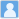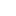• ### Predictions of nuclear charge radii based on the convolutional neural network

分类： 物理学 >> 核物理学 提交时间： 2023-09-07Cao, Yingyu Guo, Jianyou Zhou, Bo

摘要：In this study, we developed a neural network that incorporates a fully connected layer with a convolutional layer to predict the nuclear charge radii based on the relationships between four local nuclear charge radii.
The convolutional neural network (CNN) combines the isospin and pairing effects to describe the charge radii of nuclei with $A geq$ 39 and $Z geq$ 20. The developed neural network achieved a root--mean--square (RMS) deviation of 0.0195 fm for a dataset with 928 nuclei. Specifically, the CNN reproduced the trend of the inverted parabolic behavior and odd--even staggering observed in the calcium isotopic chain, demonstrating reliable predictive capability.通过